# SSC CHSL Percentage Questions with Solutions

SSC CHSL Percentage Questions with Solutions for Competitive Exams. Quantitative Aptitude (Maths) Question answer with short tricks from all shift of 2021 CHSL Exams. Very useful for upcoming SSC CGL and CHSL Exams in 2022.

## SSC CHSL Percentage Questions : 2021 (all Shifts)

Q.1: A is 150% of B and B is 40% of C. If A+B+C=20, then the value of 2B+3C – 4A is equal to:
(A) 16
(B) 15
(C) 20
(D) 14

Ans : (D) 14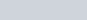$A=B\times\frac{150}{100}$
A : B = 3 : 2
B : C = 2 : 5
A : B : C = 3 : 2 : 5
A+B+C = 20
10 = 20
1 = 2
A = 6, B = 4, C = 10
so 2B+3C-4A
2 x 4 + 3 x 10 – 4 x 6
38 – 24 = 14

Q.2: One dozen notebooks quoted at 125 are available at 20% discount. How many notebooks can be bought for 75 ?
(A) 9
(B) 8
(C) 10
(D) 6

Ans : (A) 9
Printed Price = 125
Discount= 20%
Price of 12 notebooks =125-25=100
Number of notebooks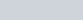$=\frac{75\times12}{100}=9$

Q.3: The population of a town increased by 15% in 2018 and 10% in 2019. Due to a pandemic, it decreased by 10% in 2020. What was the percentage increase in the population of the town in 3 years?
(A) 12.5%
(B) 17.5%
(C) 15%
(D) 13.85%

Ans : (D) 13.85%
2018 = 15% Increase
2019 = 10% Increase
2020 = 10% Decrease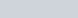$x\pm y\pm\frac{xy}{100}$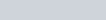$15+10+\frac{15\times10}{100}$
=26.5% Increase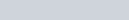$26.5-10-\frac{26.5\times10}{100}$
16.50 – 2.65=13.85%

Q.4: In a constituency, 55% of the total number of voters are males and the rest are females. If 40% of the males are illiterate and 40% of the females are literate, then by what percentage is the number of illiterate females more than that of the illiterate males (correct to one decimal place) ?
(A) 16.4%
(B) 20.8%
(C) 22.7%
(D) 21.5%

Ans : (C) 22.7%
Total voters = 100
Males= 55
Females= 45
Illiterate Males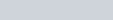$=55\times \frac{40}{100}=22$
Illiterate females$=45\times \frac{60}{100}=27$
% Increase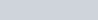$=\frac{27-22}{22}\times 100$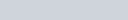$=\frac{5}{22}\times100=22.7$%

Q.5: The income of A is 40% less than that of B. If A gets 25% rise in his income and B gets 40% rise in his income, then the percentage increase (correct to two decimal places) in the combined of A and B will be:
(A) 37.86
(B) 31.67
(C) 35.19
(D) 34.38

Ans : (D) 34.38
Let B’s Income = 100
A’s Income = 60
(A+B) Total income = 160
Increase in A’s income$=60\times\frac{25}{100}=15$
A’s new income = 60+15 = 75
Similarly B’s new income =140
Thus the new income of (A+B) = 140+75=215
% Increase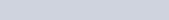$=\frac{215-160}{160}\times100 =34.38$%

Q.6: A number P is 20% more than a number Q but 10% less than a number R. What percentage is number Q of number R?
(A) 90
(B) 75
(C) 80
(D) 85

Ans : (B) 75
P : Q = [6 : 5] x 3
P : R = [9 : 10] x 2
P : Q = 18 : 15
P : R = 18 : 20
P : Q : R = 18 : 15 : 20
What percentage is number Q of number R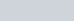$=\frac{Q}{R}\times 100$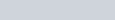$=\frac{15}{20}\times100=75$%

Q.7: A reduction of 20% in the rate of sugar enables Sudhir to get 6 kg more sugar for 960. What is the reduced rate of sugar per kg?
(A) 36
(B) 35
(C) 40
(D) 32

Ans : (D) 32
Initial Price : Reduced Price
Price ratio 5 : 4
Consumed Quantity ratio 4 : 5
1 = 6 kg
Initial quantity =6 x 4=24 kg
Reduced quantity= 6 x 5 = 30 kg
Reduced rate of sugar per kg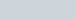$=\frac{960}{30} =32$ kg

Q.8: Two numbers are less than a third number by 37% and 30%, respectively. By what percentage is the second number more than the first?
(A)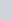$\frac{10}{9}$
(B)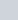$\frac{100}{9}$
(C)$\frac{100}{3}$
(D) 10

Ans : (B)$\frac{100}{9}$
I : II : III
63 : 70 : 100
Intended %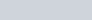$=\frac{II - I}{I}\times100$$=\frac{70-63}{63}\times100$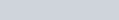$=\frac{7}{63}\times100=\frac{100}{9}$%

Q.9: Gaurav earns 800 per day. After some weeks, he earns 960 per day. What is the percentage increase in his daily earnings?
(A) 14%
(B) 18%
(C) 16%
(D) 20%

Ans : (D) 20%
Percentage increase in income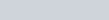$=\frac{960-800}{800}\times100$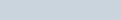$=\frac{160}{800}\times100=20$% Increase

Q.10: The average percentage of girls in class X examination in a school is 85% and that of boys is 83%. The average pass percentage of all boys and girls in class X of that school is 83.7% Find the percentage of the number of girls in class X of that school.
(A) 30%
(B) 40%
(C) 35%
(D) 45%

Ans : (C) 35%

7 : 13
% of girls$=\frac{7}{20}\times100=35$%

Q.11: A person saves 25% of his income. If his income increases by 20% and his saving remains the same, then what will be the increased percentage of his expenditure?
(A)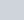$26\frac23$
(B) 26
(C) 20
(D) 30

Ans : (A)$26\frac23$
Suppose income = 100
Savings = 25बचत
Expense= 75

+ 20% Increase after

% Increase in expenditure$=\frac{95-75}{75}\times100$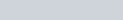$=\frac{20}{75}\times100=26\frac23$%

Q.12: Mohan’s income is 40% more than shyam’s income. Shyam’s income is what percentage less than Mohan’s income?
(A)$28\frac27$%
(B)$28\frac57$%
(C)$28\frac47$%
(D)$28\frac37$%

Ans : (C)$28\frac47$%$=\frac{40}{140}\times100=28\frac47$%

Q.13: A, B and C are three positive numbers such that A is 70% of B, and B is 40% of C. If the sum of all three numbers is 336, then 15% of the sum of B and C is:
(A) 48
(B) 44
(C) 32
(D) 42

Ans : (D) 42$A = B\times\frac{70}{100}$
A : B = 7 : 10
B : C = 2 : 5
A : B : C = 14 : 20 : 50
= 7 : 10 : 25
42 = 336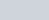$1 =\frac{336}{42}$
1 = 8
B+C = 80+200=280$=280\times\frac{15}{100}=42$

Q.14: Renu spends 68% of her income. When her income increases by 40%, she increases her expenditure by 30%. Her savings are increased by:
(A) 37.98%
(B) 62.5%
(C) 61.25%
(D) 51.6%

Ans : (C) 61.25%

+30% Increase after

% increase in savings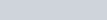$=\frac{51.6 - 32}{32}\times100$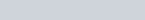$=\frac{19.6}{32}\times100 = 61.25$%

Q.15: A’s salary is 15% less than B’s salary. B’s salary is 30% less than C’s salary. By how much percent approximately, is C’s salary more than A’s salary?
(A) 75
(B) 45
(C) 68
(D) 40

Ans : (C) 68
A : B = 17 : 20
B : C = 7 : 10
Ratio of salary of A : B : C
= 119 : 140 : 200
According to question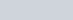$\frac{C - A}{A}\times100$$=\frac{200-119}{119}\times100=68.06$%
=68%

Q.16: Two numbers A and B are in the ratio 13 : 17. If A is increased by 15% and B is increased by 30%, then the new ratio of A to B will be:
(A) 21 : 31
(B) 23 : 33
(C) 23 : 34
(D) 21 : 29

Ans : (C) 23 : 34
A : B
13 : 17
According of question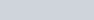$\frac{13\times115}{100} : \frac{17\times130}{100}$
A : B = 23 : 34

Thanks for study the SSC CHSL Percentage Questions (English).

Percentage Questions in Hindi with Solutions – SSC CHSL Exam

## Maths Practice Set for Competitive Exams

Simple and Compound Interest Questions with Answers

Time and Work Questions with Answers